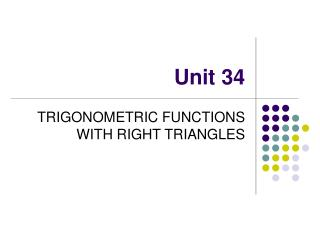DownloadDownload PresentationUnit 34

# Unit 34

Download Presentation## Unit 34

- - - - - - - - - - - - - - - - - - - - - - - - - - - E N D - - - - - - - - - - - - - - - - - - - - - - - - - - -
##### Presentation Transcript

1. Unit 34 TRIGONOMETRIC FUNCTIONS WITH RIGHT TRIANGLES

2. VARIATION OF FUNCTIONS • As the size of an angle increases, the sine, tangent, and secant functions increase, but the cofunctions (cosine, cotangent, cosecant) decrease • Which is greater: cos 38° or cos 43°? • Since the cosine function decreases as the size of the angle increases, cos 38° is greater than cos 43° • Which is greater: tan 42° or tan 24°? • Since the tangent function increases as the size of the angle increases, tan 42° is greater than tan 24°

3. sin A = cos (90° – A) cos A = sin (90° – A) tan A = cot (90° – A) cot A = tan (90° – A) sec A = csc (90° – A) csc A = sec (90° – A) FUNCTIONS OF COMPLEMENTARY ANGLES • Two angles are complementary when their sum is 90°. For example, 30° is the complement of 60°, and 60° is the complement of 30° • A function of an angle is equal to the cofunction of the complement of the angle

4. FINDING UNKNOWN ANGLES • Procedure for determining an unknown angle when two sides are given: • In relation to the desired angle, identify two given sides as adjacent, opposite, or hypotenuse • Determine the functions that are ratios of the sides identified in relation to the desired angle • Choose one of the two functions, substitute the given sides in the ratio • Determine the angle that corresponds to the quotient of the ratio

5. B 3.2" 5.7" EXAMPLE OF FINDING AN ANGLE • Determine B to the nearest tenth of a degree in the triangle below:

6. EXAMPLE (Cont) • Since 5.7" is opposite B and 3.2" is adjacent B; either the tangent or the cotangent function could be used • Remember that when looking for an angle you will use arctan in this case or tan-1 on your calculator • Choosing the tangent function:

7. FINDING UNKNOWN SIDES • Procedure for determining an unknown side when an angle and a side are given: • In relation to the given angle, identify the given side and the unknown side as adjacent, opposite, or hypotenuse • Determine the trigonometric functions that are ratios of the sides identified in relation to the given angle • Choose one of the two functions and substitute the given side and given angle • Solve as a proportion for the unknown side

8. x 46.3° 2.7 cm EXAMPLE OF FINDING A SIDE • Determine side x (to the nearest hundredth) of the right triangle shown below:

9. EXAMPLE (Cont) • In relation to the 46.3° angle, the 2.7 cm side is the adjacent side and side x is the hypotenuse. Thus, either the cosine or the secant function could be used • Choosing the cosine function: so x = 3.91 cm Ans

10. PRACTICE PROBLEMS • Which is greater: sin 48° or sin 32°? • Which is greater: csc 54.3° or csc 45.3°? • What is the cofunction of the complement of sec 35°? • What is the cofunction of the complement of cos 82°?

11. A 4.4" 3.2" PRACTICE PROBLEMS (Cont) • Determine angle A to the nearest tenth of a degree in the triangle shown below:

12. b 28° 5.4 mm PRACTICE PROBLEMS (cont) • Determine side b in the triangle given below. Round your answer to two decimal places. • Determine 1 (to the nearest tenth of a degree) in the triangle given below. 5.86" 1 3.25"

13. PROBLEM ANSWER KEY • sin 48° • csc 45.3° • csc 55° • sin 8° • 46.7° • 4.77 mm • 33.7°